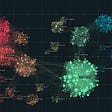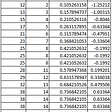# Short Introduction to ROC curve

The Returning Operating Characteristic(ROC) curve, in short, shows the tradeoff between the True Positive Rate(TPR) and False Positive Rate(FPR). It’s a useful relational plot to evaluate a model’s “goodness” for a binary classification problem. The Area Under the Curve(AUC) summarizes the ROC curve to a single metric. The AUC score differs from other metrics such as precision, recall, and accuracy because the AUC score grades the classifier across all possible thresholds.

Blog Outline:

1. TPR/FPR
2. Threshold
3. ROC curve

## TPR/FPR

The TPR and FPR are calculated with the number of occurrences of True Negatives(TN), False Positives(FP), False Negatives(FN), and True Positives(TP).

Correct(Truth):
TP = Ground Truth 1 / Predicted 1
TN = Ground Truth 0 / Predicted 0

Incorrect(False):
FP = Ground Truth 0 / Predicted 1
FN = Ground Truth 1 / Predicted 0

The TPR and FPR will differ depending on the set threshold value.

## Threshold

A threshold is simply a cutoff for the model’s output. The output for a binary classifier is a probability, and if the output is higher than the threshold(for example, 0.50), it will be assigned to 1, else 0.

If the threshold was to be set at 0.00, then the classifier will predict every observation as 1. This will therefore result FPR and TPR to be 1 because there will be no negatives, regardless of true or not(FP / (FP + 0) = 1 and TP / (TP + 0) = 1).

If threshold was to be set at 1.00, then every observation will be predicted as 0 (0 / (0 + TN) = 0 and 0 / (0 + FN) = 0) and therefore the TPR and FPR will be 0.

## ROC Curve

The ROC curve is an interpolated line of TPR and FPR values for a range of possible thresholds. It highlights the tradeoff between TPR and FPR, and the AUC can be calculated as a metric of “goodness” for the classifier.

IMPORTANT NOTE: The values of “threshold” are not scaled appropriately with the X-axis “False Positive Rate.” The False Positive Rate at 0.50 DOES NOT equate to the threshold value of 0.50.

The ROC curve is dependant on the threshold; however, it only highlights the relation between TPR and FPR. I added the purple threshold axis to show that each threshold value would return two values (TPR, FPR), which can then be plotted for a ROC curve.

Caution: Using AUC as an evaluation for a model dealing with large class imbalance might be optimistically deceptive. For example, if the observations of class = 0 were overwhelmingly larger than class = 1, the FPR would be consistently lower than TPR.

--

--

--

## More from Albert Um

Hello! My name is Albert Um.

Love podcasts or audiobooks? Learn on the go with our new app.

## The Three Types of A/B Tests## Mathematical Recipe of Dimensionality Reduction## Scrape Company Profile Page on Linkedin in python## An Introduction to Board Application## An Intro to Pybaseball’s Statcast## How to Become a Successful Data Storyteller## How I collected an Arabic DataSet of more than 5000 articles?## What cyber security can teach us about COVID-19 testing## Albert Um

Hello! My name is Albert Um.

## What is the Difference Between Hierarchical and Partitional Clustering## Q-Q Plots — A view from statistics perspective## Assumptions of Linear Regression (with Python Implementation)## Can robots be sommeliers?